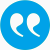Frühwirth-Schnatter, Sylvia. 2001. Markov chain Monte Carlo estimation of classical and dynamic switching and mixture Models. Journal of the American Statistical Association 96 194-209.

BibTeX

Abstract

Bayesian estimation of a very general model class, where the distribution of the observations depends on a latent process taking values in a discrete state space, is discussed in this article. This model class covers finite mixture modeling, Markov switching autoregressive modeling, and dynamic linear models with switching. The consequences the unidentifiability of this type of model has on Markov chain Monte Carlo (MCMC) estimation are explicitly dealt with. Joint Bayesian estimation of all latent variables, model parameters, and parameters that determine the probability law of the latent process is carried out by a new MCMC method called permutation sampling. The permutation sampler first samples from the unconstrained posterior-which often can be done in a convenient multimove manner-and then applies a permutation of the current labeling of the states of the latent process. In a first run, the random permutation sampler used selected the permutation randomly. The MCMC output of the random permutation sampler is explored to find suitable identifiability constraints. In a second run, the permutation sampler was used to sample from the constrained posterior by imposing identifiablity constraints. This time a suitable permutation is applied if the identifiability constraint is violated. For illustration, two detailed case studies are presented, namely finite mixture modeling of fetal lamb data and Markov switching autoregressive modeling of the U.S. quarterly real gross national product data.

Tags

Press 'enter' for creating the tag

Publication's profile

Status of publication Published WU Journal article Journal of the American Statistical Association SCI A FIN-A, VW-A, WH-A English Markov chain Monte Carlo estimation of classical and dynamic switching and mixture Models 96 2001 194 209 http://www.jstor.org/stable/info/2670359

Associations

People
Frühwirth-Schnatter, Sylvia (Details)
Organization
Institute for Statistics and Mathematics IN (Details)